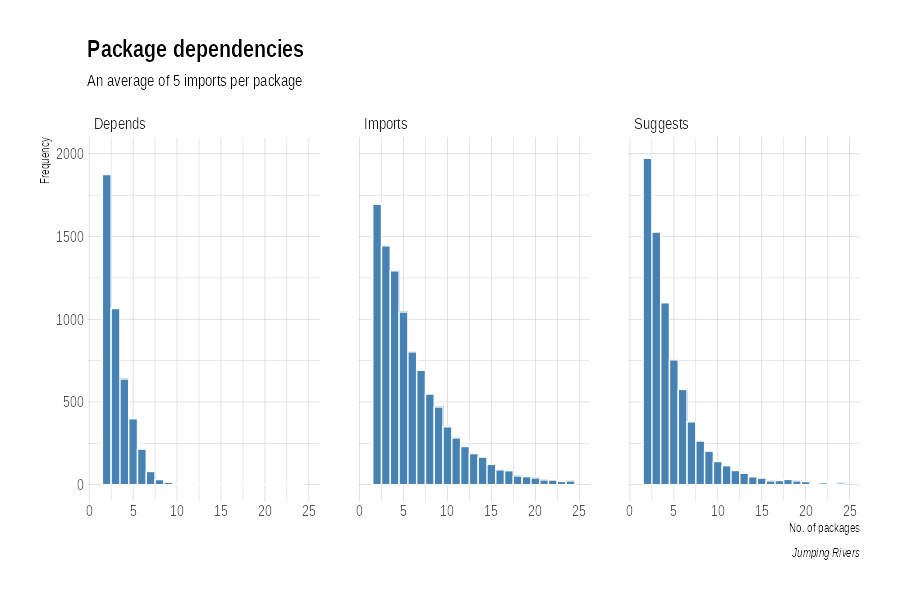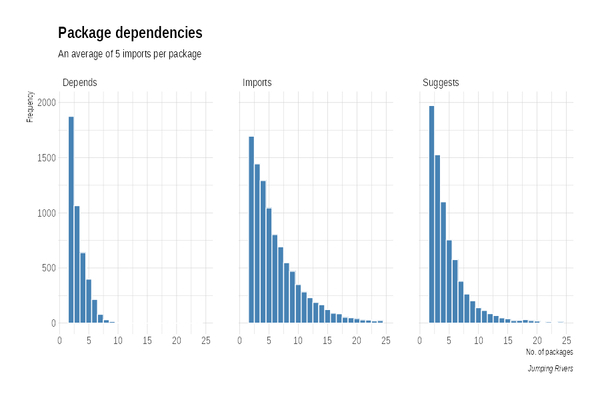GitHub

# What R version do you really need for a package?

Published: November 1, 2018At Jumping Rivers we run a lot of R courses. Some of our most popular courses revolve around the tidyverse, in particular, our Introduction to the tidyverse and our more advanced mastering course. We even trained over 200 data scientists NHS - see our case study for more details.

As you can imagine, when giving an on-site course, a reasonable question is what version of R is required for the course. We always have an RStudio cloud back-up, but it’s nice for participants to run code on their own laptop. If participants are to bring there own laptop it’s trivial for them to update R. But many of our clients are financial institutions or government where an upgrade is a non-trivial process.

So, what version of R is required for a tidyverse course? For the purposes of this blog post, we will define the list of packages we are interested in as

``````library("tidyverse")
tidy_pkgs = c("ggplot2", "purrr", "tibble", "dplyr", "tidyr", "stringr",
``````

The code below will work with any packages of interest. In fact, you can set `pkgs` to all R packages in CRAN, it just takes a while.

## Package descriptions

In R, there is a handy function called `available.packages()` that returns a matrix of details corresponding to packages currently available at one or more repositories. Unfortunately, the format isn’t initially amenable to manipulation. For example, consider the {readr} package

``````readr_desc = available.packages() %>%
as_tibble() %>%
``````

I immediately converted the data to a tibble, as that

• changed the rownames to a proper column
• changed the matrix to a data frame/tibble, which made selecting easier

Looking at the `read_desc`, we see that it has a minimum R version

``````readr_desc\$Depends
##  "R (>= 3.0.2)"
``````

but due to the format, it would be difficult to compare to R versions. Also, the list of imports

``````readr_desc\$Imports
##  "Rcpp (>= 0.12.0.5), tibble, hms, R6"
``````

has a similar problem. For example, with the data in this format, it would be difficult to select packages that depend on {tibble}.

## Tidy package descriptions

We currently have four columns

• Imports, Depends, Suggests, Enhances

each entry in these columns contains multiple packages, with possible version numbers. To tidy the data set I’m going to create four new columns:

• `depend_type`: one of Imports, Depends, Suggests, Enhances and LinkingTo
• `depend_package`: the package name
• `depend_version`: the package version
• `depend_condition`: something like equal to, less than or greater than

The hard work is done by the function `clean_dependencies()`, which is at the end of the blog post. It essentially just does a bit of string manipulation to separate out the columns. The function works per package, so we iterate over packages using `map_df()`

``````pkg_deps = c("Depends", "Enhances", "Suggests", "Imports", "LinkingTo")
av_pkgs = available.packages() %>%
as_tibble()

av_pkgs = map_df(pkg_deps, clean_dependencies, av_pkgs = av_pkgs) %>%
select(-pkg_deps)

# Std version: 3.4 -> 3.4.0
av_pkgs = av_pkgs %>%
mutate(depend_version = if_else(depend_package == "R",
clean_r_ver(depend_version),
depend_version))

# Standardise column names
colnames(av_pkgs) = str_to_lower(colnames(av_pkgs))
``````

After this step, we now have tibble with tidy columns:

``````av_pkgs %>%
select(package, depend_type, depend_package, depend_version, depend_condition) %>%
slice(1:4)
## # A tibble: 4 x 5
##   package  depend_type depend_package depend_version depend_condition
##   <chr>    <chr>       <chr>          <chr>          <chr>
## 1 A3       Depends     R              2.15.0         >=
## 2 abbyyR   Depends     R              3.2.0          >=
## 3 abc      Depends     R              2.10.0         >=
## 4 abc.data Depends     R              2.10.0         >=
``````

and we can see minimum R version the package authors have indicated for their package. However, this isn’t the minimum version required. Each package imports a number of other packages, e.g. the {readr} imports 4 packages

``````av_pkgs %>%
filter(package == "readr" & depend_type == "Imports") %>%
select(depend_package) %>%
pull()
##  "Rcpp"   "tibble" "hms"    "R6"
``````

and each of those packages, also import other packages. Clearly, the minimum version required to install {dplyr} is the maximum R version of all imported packages

### Some interesting things

Before we work out the maximum R version for each set of imports, we should first investigate how many imports each package using a bit of {dplyr}

``````imp = av_pkgs %>%
group_by(package, depend_type) %>%
summarise(n = length(depend_package)) %>%
arrange(package, n) %>%
filter(depend_type != "Enhances" & depend_type != "LinkingTo")

imp %>%
group_by(depend_type) %>%
summarise(mean(n))
## # A tibble: 3 x 2
##   depend_type `mean(n)`
##   <chr>           <dbl>
## 1 Depends          1.96
## 2 Imports          4.98
## 3 Suggests         3.63
``````

Using histograms we get a better idea of the overall numbers. Note that here we’re using the ipsum theme from the {hrbrthemes} package.

``````library(hrbrthemes)
ggplot(imp, aes(n)) +
geom_histogram(binwidth = 1, colour= "white", fill = "steelblue") +
facet_wrap(~depend_type) +
xlim(c(0, 25)) + ylim(c(0, 6000)) +
theme_ipsum() +
labs(x="No. of packages", y="Frequency",
title="Package dependencies",
subtitle="An average of 5 imports per package",
caption="Jumping Rivers")
``````## Maximum overall imports

As I’ve mentioned, we need to obtain not just the imported packages, but also their dependencies. Fortunately, the {tools} package comes to our rescue,

``````tools::package_dependencies("readr",
recursive = TRUE) %>%
unlist() %>%
unname()
##   "Rcpp"       "tibble"     "hms"        "R6"         "BH"
##   "methods"    "pkgconfig"  "rlang"      "utils"      "cli"
##  "crayon"     "pillar"     "assertthat" "grDevices"  "fansi"
##  "utf8"       "tools"
``````

Using the `package_dependencies()` function, we simply

• Obtain a list of dependencies for a given package
• Extract the maximum version of R for all packages in the list

At the end of this post, there are two helper functions:

• `max_r_version()` - takes a vector of R versions, and returns a maximum version. E.g.
``````max_r_version(c("3.2.0", "3.3.2", "3.2.0"))

## 3.3.2
``````
• `get_r_ver()` - calls `package_dependencies()` and returns the maximum R version out of all of the dependencies.

Also, we have simplified some the details and what we’ve done isn’t quite right - it’s more of a first approximation. See the end of the post for details

Now that’s done, we can pass the list of {tidyverse} packages then compare their stated R version, with the actual required R version

``````# Here we are using just the tidyverse
# But this works with any packages
tidy = map_dfr(tidy_pkgs, get_r_ver, av_pkgs)  %>%
group_by(package) %>%
slice(1) %>%
select(package, r_real, r_cur)
``````

We then select the packages where there is a difference

``````tidy %>%
filter(r_real != r_cur | (!is.na(r_real) & is.na(r_cur)))
# A tibble: 3 x 3
# Groups:   package 
package   r_real r_cur
<chr>     <chr>  <chr>
2 tidyr     3.1.2  3.1.0
``````

The largest difference in R versions is for {readr} (which feeds into the {tidyverse}). {readr} claims to only need R version 3.0.2 but a bit more investigation shows that {readr} depends on the tibble package which is version 3.1.0. Although, it is worth noting that 3.1.0 is fairly old!

## Take away lessons

The takeaway message is that dependencies matter. A single change affects everything in the package dependency tree. The other lesson is that the tidyverse team have been very careful about there dependencies. In fact, all of their packages are checked on R 3.1, 3.2, …, devel

## Simplifications: skipping package versions

In this analysis, we’ve completely ignored version numbers and always assumed we need the latest version of a package. This clearly isn’t correct. So to do this analysis properly, we would need the historical DESCRIPTION files for packages and use that to determine versions.

Thanks to Jim Hester who spotted an error in a previous version of this post.### Functions

``````clean_dependencies = function(av_pkgs, type) {

no_of_cols = max(str_count(av_pkgs[[type]], ","), na.rm = TRUE) + 1
cols = paste0(type, seq_len(no_of_cols))
av_clean = av_pkgs %>%
separate(type, sep = ",", into = cols, fill = "right", remove = FALSE) %>%
gather(key = type, pkg, cols)  %>%
drop_na(pkg) %>%
mutate(type = !!type) %>%
separate(pkg, sep = "\\(", into = c("package", "version"), fill = "right") %>%
mutate(version = str_remove(version, "\\)"),
package = str_remove(package, "\\)")) %>%
mutate(version = str_remove(version, "\n"),
package = str_remove(package, "\n")) %>%
mutate(version = str_trim(version),
package = str_trim(package)) %>%
filter(package != "")

## Detect where version is
version_loc = str_locate(av_clean\$version, "[0-9]")
av_clean %>%
mutate(condition = str_sub(version, end = version_loc[, "end"] - 1),
version = str_sub(version, start = version_loc[, "end"])) %>%
rename(depend_type = type,
depend_package = package,
depend_version = version,
depend_condition = condition) %>%
mutate(depend_condition = str_trim(depend_condition))
}

clean_r_ver = function(vers) {
nas = is.na(vers)
vers[!nas] = paste0(vers[!nas], if_else(str_count(vers[!nas], "\\.") == 2, "", ".0"))
vers
}

max_r_version = function(r_versions) {
s = str_split(r_versions, "\\.", n = 3)
major = map(s, `[`, 1) %>%
map(as.integer)
if(sum(major == 3) > 0) {
maj_number = 3
} else if(sum(major == 2) > 0) {
maj_number = 2
} else if(sum(major == 1) > 0) {
maj_number = 1
} else {
return("")
}

minor = map(s, `[`, 2) %>%
map(as.integer)

min_number= minor[which(major == maj_number)] %>%
unlist() %>%
max()
third = map(s, `[`, 3) %>%
map(as.integer)

third_number = third[which(major == maj_number & minor == min_number)] %>%
unlist() %>%
max()
glue::glue("{maj_number}.{min_number}.{third_number}")
}

get_r_ver = function(pkg, clean_av) {

r_cur = clean_av %>%
filter(depend_package == "R" & package == pkg) %>%
select(depend_version) %>%
pull()

if(length(r_cur) == 0) r_cur = NA
pkg_dep = tools::package_dependencies(pkg,
recursive = TRUE) %>%
unlist() %>%
unname() %>%
c(pkg)
# Optional and recommended packages
with_r = filter(av_pkgs, !is.na(priority)) %>%
select(package) %>% pull() %>%
unique()

pkg_dep = pkg_dep[!(pkg_dep %in% with_r)]

r_ver = clean_av %>%
filter(package %in% pkg_dep) %>%
filter(depend_type == "Depends") %>%
filter(depend_package == "R") %>%
select(depend_version) %>%
summarise(max_r_version(depend_version)) %>%
pull()

clean_av %>%
filter(package == pkg) %>%
mutate(r_real = as.character(r_ver),
r_cur = r_cur)

}
``````